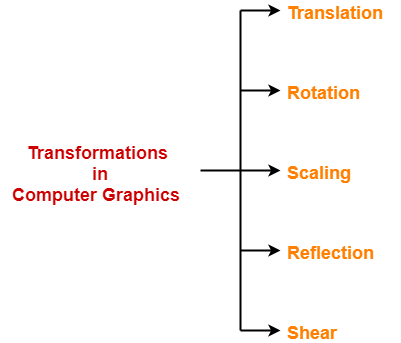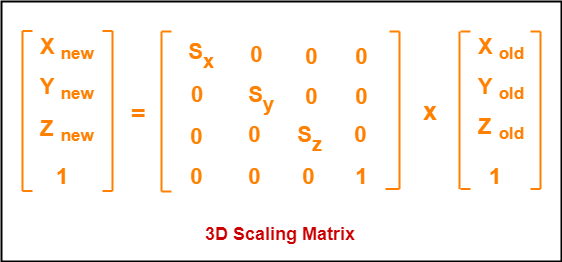# 3D Scaling in Computer Graphics | Definition | Examples

## 3D Transformations in Computer Graphics-

We have discussed-

• Transformation is a process of modifying and re-positioning the existing graphics.
• 3D Transformations take place in a three dimensional plane.

In computer graphics, various transformation techniques are-## 3D Scaling in Computer Graphics-

 In computer graphics, scaling is a process of modifying or altering the size of objects.

• Scaling may be used to increase or reduce the size of object.
• Scaling subjects the coordinate points of the original object to change.
• Scaling factor determines whether the object size is to be increased or reduced.
• If scaling factor > 1, then the object size is increased.
• If scaling factor < 1, then the object size is reduced.

Consider a point object O has to be scaled in a 3D plane.

Let-

• Initial coordinates of the object O = (Xold, Yold,Zold)
• Scaling factor for X-axis = Sx
• Scaling factor for Y-axis = Sy
• Scaling factor for Z-axis = Sz
• New coordinates of the object O after scaling = (Xnew, Ynew, Znew)

This scaling is achieved by using the following scaling equations-

• Xnew = Xold x Sx
• Ynew = Yold x Sy
• Znew = Zold x Sz

In Matrix form, the above scaling equations may be represented as-## Problem-01:

Given a 3D object with coordinate points A(0, 3, 3), B(3, 3, 6), C(3, 0, 1), D(0, 0, 0). Apply the scaling parameter 2 towards X axis, 3 towards Y axis and 3 towards Z axis and obtain the new coordinates of the object.

## Solution-

Given-

• Old coordinates of the object  = A (0, 3, 3), B(3, 3, 6), C(3, 0, 1), D(0, 0, 0)
• Scaling factor along X axis = 2
• Scaling factor along Y axis = 3
• Scaling factor along Z axis = 3

### For Coordinates A(0, 3, 3)

Let the new coordinates of A after scaling = (Xnew, Ynew, Znew).

Applying the scaling equations, we have-

• Xnew = Xold x Sx = 0  x 2 = 0
• Ynew = Yold x Sy = 3 x 3 = 9
• Znew = Zold x Sz = 3 x 3 = 9

Thus, New coordinates of corner A after scaling = (0, 9, 9).

### For Coordinates B(3, 3, 6)

Let the new coordinates of B after scaling = (Xnew, Ynew, Znew).

Applying the scaling equations, we have-

• Xnew = Xold x Sx = 3  x 2 = 6
• Ynew = Yold x Sy = 3 x 3 = 9
• Znew = Zold x Sz = 6 x 3 = 18

Thus, New coordinates of corner B after scaling = (6, 9, 18).

### For Coordinates C(3, 0, 1)

Let the new coordinates of C after scaling = (Xnew, Ynew, Znew).

Applying the scaling equations, we have-

• Xnew = Xold x Sx = 3  x 2 = 6
• Ynew = Yold x Sy = 0 x 3 = 0
• Znew = Zold x Sz = 1 x 3 = 3

Thus, New coordinates of corner C after scaling = (6, 0, 3).

### For Coordinates D(0, 0, 0)

Let the new coordinates of D after scaling = (Xnew, Ynew, Znew).

Applying the scaling equations, we have-

• Xnew = Xold x Sx = 0  x 2 = 0
• Ynew = Yold x Sy = 0 x 3 = 0
• Znew = Zold x Sz = 0 x 3 = 0

Thus, New coordinates of corner D after scaling = (0, 0, 0).

To gain better understanding about 3D Scaling in Computer Graphics,

Watch this Video Lecture

Next Article- 3D Reflection in Computer Graphics

Get more notes and other study material of Computer Graphics.

Watch video lectures by visiting our YouTube channel LearnVidFun.

SummaryArticle Name
3D Scaling in Computer Graphics | Definition | Examples
Description
3D Scaling in Computer Graphics is a process of altering the size of objects in 3D plane. Scaling in Computer Graphics Definition, Solved Examples and Problems.
Author
Publisher Name
Gate Vidyalay
Publisher Logo